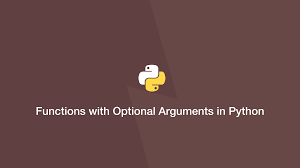# How to Create Python Function with Optional Arguments

Sometimes you may need to create python function with optional arguments. In this article, we will learn how to do this using *args, **kwargs and using default argument values.

## How to Create Python Function with Optional Arguments

Python provides two ways to provide optional arguments in python – *args and **kwargs. We will also look at couple of unusual ways to define optional argument in python functions.

## 1. Using *args

In this case, you can simply add *args after the required arguments of your function definition. Here is a simple example of function that accepts two mandatory arguments a & b, and allows optional arguments.

```def myfunc(a,b, *args):
for ar in args:
print ar
myfunc(a,b,c,d,e,f)```

In the above function, the optional arguments c,d,e,f are stored in args as tuple items. You can loop through the args tuple to access the optional arguments. So the above function will simply print all optional arguments.

Please note, *args must be specified after all the mandatory arguments of a function. Otherwise, even required arguments will be stored in it.

If you are not sure about the number of arguments you need to pass to your function, you can use *args to capture optional arguments.

In fact, you can use *args to not only define optional arguments but also call them. Here is an example of function that uses two required arguments but we use *args tuple to specify input arguments. We will define a tuple which contains all the input variables required for the function.

```def myfunc(a,b):
print a,b
args=(1,2)
myfunc(*args)
output: 1 2
```

## 2. Using **kwargs

You can also use **kwargs to handle optional arguments, if you want to name your optional arguments. In this case, they will be keyword arguments, that is, named arguments, which are stored as a python dictionary kwargs.

```def myfunc(a,b, **kwargs):
c = kwargs.get('c', None)
d = kwargs.get('d', None)

myfunc(a,b, c='cat', d='dog', ...)```

In the above function, whatever variable you pass as keyword argument or optional argument, it is used as a key to create a key-value pair in dictionary kwargs. The variable’s value is used as the key’s value. For example, in above example, kwargs = {‘c’:’cat’, ‘d’:’dog’}

You can not only use **kwargs to specify optional arguments in function definition, but also use it in function call. Here is an example for it. In this case, you need to define a dictionary with function arguments.

```def myfunc(a,b):
print a,b
kwargs={'a':1,'b':2}
myfunc(**kwargs)
output: 1 2
```

## 3. Using default Value

You can also set a default value for an argument, to make it optional. Here is an example function which uses a & b as compulsory arguments, and c as optional argument.

```def f(a,b, c = "1"):
print(a,b,c)

f("a",'b')

output: "a",'b',1```

## 4. Using both *args & **kwargs

You can always use both *args & **kwargs at the same time. Here is an example.

```def myFun(*args,**kwargs):
print("args: ", args)
print("kwargs: ", kwargs)

# Now we can use both *args ,**kwargs
# to pass arguments to this function :
myFun('a','for','apple',first="b",mid="for",last="ball")

output:
args: ('a', 'for', 'apple')
kwargs {'first': 'b', 'mid': 'for', 'last': 'ball'}```

In this case, it is important to note that all named arguments (first, mid, last) in function will be saved to kwargs while unnamed arguments will be saved in args.

In this article, we have learnt several different ways to work with optional arguments in python.